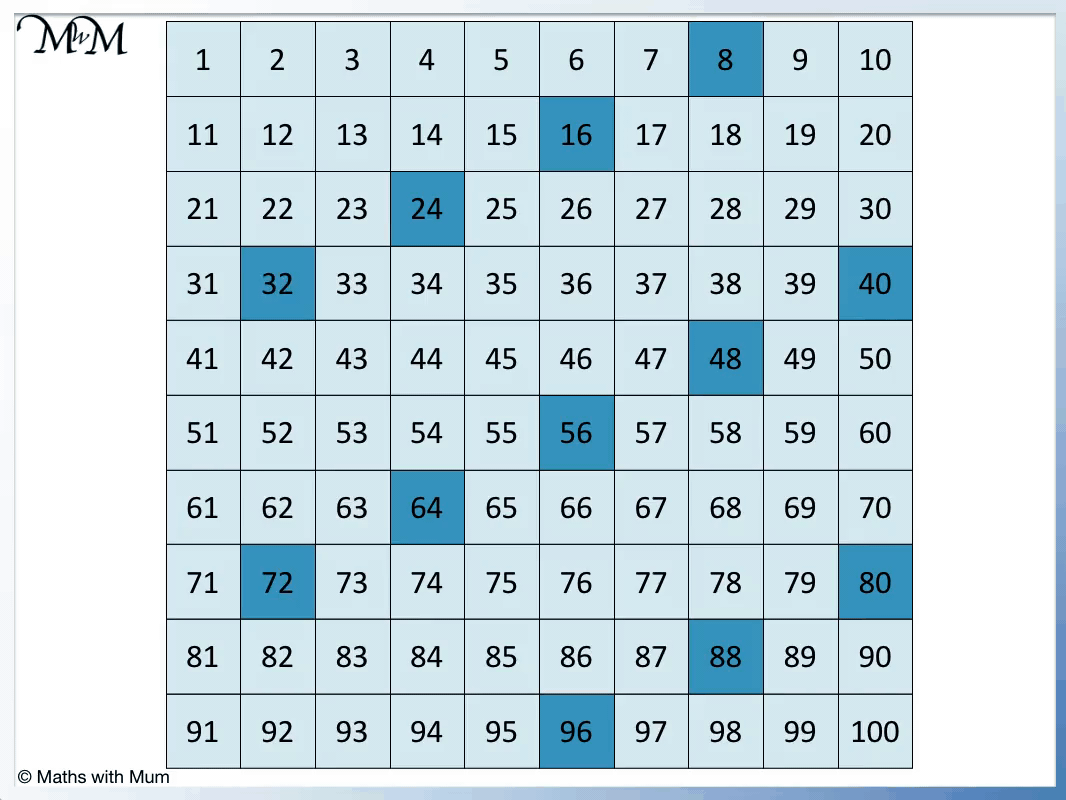# Multiples of 8

Multiples of 8• The multiples of 8 are numbers that can be divided exactly by 8, leaving no remainder.
• The first few multiples of 8 are the numbers in the 8 times table.
• For example 2 × 8 = 16 and so, the second multiple of eight is 16.
• For example 100 × 8 = 800 and so, the hundredth multiple of 8 is 800.
• A number is a multiple of 8 if it can be halved, halved and halved again to leave a whole number.
• For example, 24 is a multiple of 8 because half of 24 is 12, half of 12 is 6 and half of 6 is 3.

A multiple of 8 is any number that can be divided by 8 exactly, leaving no remainder.#### Multiples of 8 Flashcards

Click on the multiples of 8 flashcards below to memorise the 8 times table:

×
×

=#### Multiples of 8: Interactive Questions

Multiples of 8: Interactive Questions

# Multiples of 8

## What are Multiples of 8?

Multiples of 8 are numbers that can be divided exactly by 8. Multiples of 8 are found by counting up in eights. The first few multiples of 8 are: 8, 16, 24, 32, 40, 48, 56, 64, 72, 80, 88 and 96.

The first few multiples of 8 are the numbers in the 8 times table. Here is a list of the first twelve multiples of 8:To teach the multiples of 8, place the numbers on a number grid. We can count on in eights from one number to the next. Here are the multiples of 8 shown on the chart:To learn the multiples of 8, it can help to look for patterns in the positions of the numbers on the chart.

We can see that the multiples of 8 form diagonal patterns on the chart.

Multiples of 8 always end in 0, 2, 4, 6 and 8. Multiples of 8 are always even numbers.To test if a number is a multiple of 8, half it three times. If the result is a whole number, the number is a multiple of 8. If the result is not a whole number, the number is not a multiple of 8.

For example, we can halve 88 three times in a row. Half of 88 is 44, half of 44 is 22 and half of 22 is 11. 11 is a whole number and so, 88 is a multiple of 8.

20 is not a multiple of 8. It cannot be halved three times to leave a whole number. For example, half of 20 is 10, half of 10 is 5 but half of 5 is 2.5. 2.5 is not a whole number and so, 20 is not a multiple of 8.

All multiples of 8 are also multiples of 4. This is because 4 divides exactly into 8.

## How do you Find Multiples of 8?

To find multiples of 8, multiply any whole number by 8. For example, the tenth multiple of 8 is 80 because 10 × 8 = 80. Alternatively, multiples of 8 can be found by starting at zero and counting up in eights.

We can start at zero and count up in eights to find the multiples of 8.

0 + 8 = 8, 8 + 8 = 16, 16 + 8 = 24, 24 + 8 = 32, 32 + 8 = 40 and so on.

Here are the multiples of 8 to 100.There are infinite multiples of 8.

We can continue to add on 8 to find further multiples of 8.

96 + 8 = 104, 104 + 8 = 112 and so on.

## Multiples of 8 up to 100

There are 12 multiples of 8 that are less than 100. They are:

8, 16, 24, 32, 40, 48, 56, 64, 72, 80, 88 and 96.

Here is a longer list of multiples of 8:

8, 16, 24, 32, 40, 48, 56, 64, 72, 80, 88, 96, 104, 112, 120, 128, 136, 144, 152, 160, 168, 176, 184, 192, 200, 208, 216, 224, 232, 240, 248, 256, 264, 272, 280, 288, 296, 304, 312, 320, 328, 336, 344, 352, 360, 368, 376, 384, 392, 400.Now try our lesson on Lattice Multiplication Method where we learn how to use the lattice method for multiplying numbers.error: Content is protected !!